In Online Education NCERT Solutions for Class 7 Maths Chapter 11 Perimeter and Area Ex 11.4 are part of NCERT Solutions for Class 7 Maths. Here we have given NCERT Solutions for Class 7 Maths Chapter 11 Perimeter and Area Ex 11.4.

 Board CBSE Textbook NCERT Class Class 7 Subject Maths Chapter Chapter 11 Chapter Name Perimeter and Area Exercise Ex 11.4 Number of Questions Solved 11 Category NCERT Solutions

## Online Education NCERT Solutions for Class 7 Maths Chapter 11 Perimeter and Area Ex 11.4

Question 1.
A garden is 90 m long and 75 m broad. A path 5 m wide is to be built outside and around it. Find the area of the path. Also, find the area of the garden in a hectare.
Solution: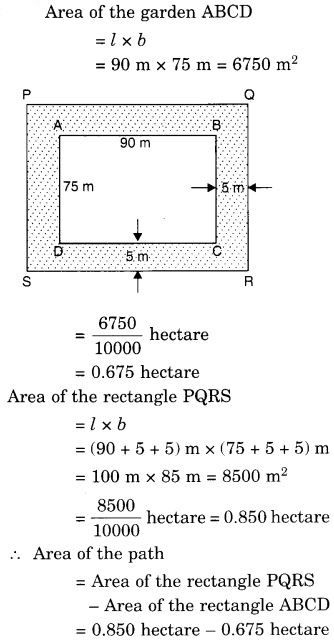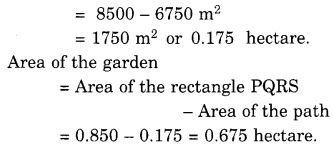Question 2.
A 3 m wide path runs outside and around a rectangular park of length 125 m and breadth 65 m. Find the area of the path.
Solution:
PQ = 125 m + 3m + 3m = 131 m
QR = 65m + 3m + 3m = 71 m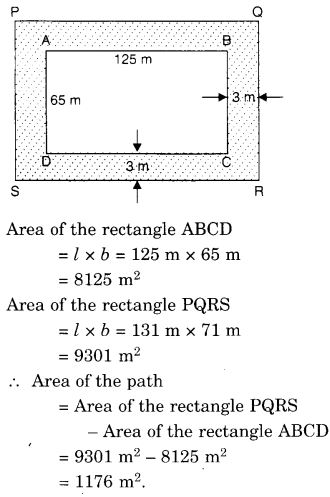Question 3.
A picture is painted on a cardboard 8 cm long and 5 cm wide such that there is a margin of 1.5 cm along each of its sides. Find the total area of the margin.
Solution: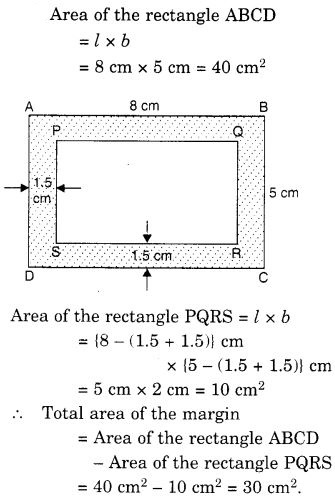Question 4.
A verandah of width 2.25 m is constructed all along the outside of a room which is 5.5 m long and 4 m wide. Find:
(i) the area of the verandah
(ii) the cost of cementing the floor of the verandah at the rate of ₹200 per m2.
Solution: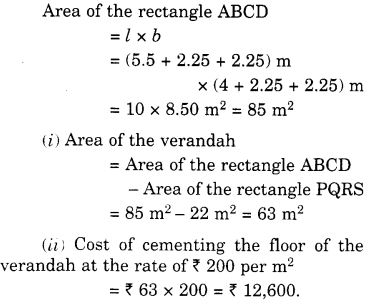Question 5.
A path 1 rn wide is built along the border and inside a square garden of side 30 m. Find.
(i) the area of the path.
(ii) the cost of planting grass in the remaining portion of the garden at the rate of f 40 per m2.
Solution: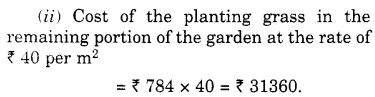Question 6.
Two crossroads, each of width 10 m, cut at right angles through the centre of a rectangular park of the length 700 m and the breadth 300 m parallel to its sides. Find the area of the roads. Also, find the area of the park excluding crossroads. Give the answer in hectares.
Solution: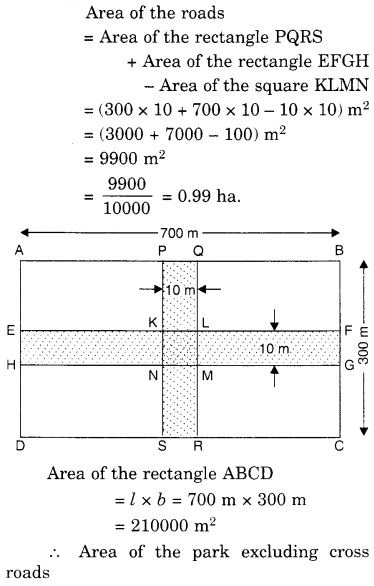Question 7.
Through a rectangular field of length 90 m and breadth 60 m, two roads are constructed which are parallel to the sides and cut each other at right angles through the centre of the fields. If the width of each road is 3 m, find
(i) the area covered by the roads.
(ii) the cost of constructing the roads at the rate of ₹ 110 per m2.
Solution:
(i) Area covered by the roads = Area of the rectangle PQRS + Area of the rectangle EFGH – Area of the square KLMN
= (90 × 3 + 60 × 3 – 3 × 3) m2
= (270 + 180 – 9) m2
= 441 m2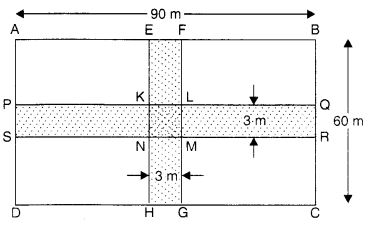(ii) Cost of constructing the roads at the rate of ₹ 110 per m2 ₹ 441 × 110 = ₹ 48510.

Question 8.
Pragya wrapped a cord around the circular pipe of the radius 4 cm (adjoining figure) and cut off the length required of the cord. Then she wrapped it around the square box of the side 4 cm (also shown. Did she hate any cord left? = ( π = 3.14)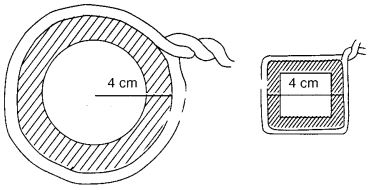Solution:
Radius of the circular pipe (r) = 4 cm
∴ Circumference of the circular pipe = 2πr = 2 × 3.14 × 4 cm = 25.12 cm
Perimeter of the square box of the side 4 cm = 4 × side = 4 × 4 cm = 16 cm
∵ 25.12 cm > 16 cm
∴ She had extra cord left and the length of the cord left = (25.12 – 16) cm = 9.12 cm.

Question 9.
The following figure represents a rectangular lawn with a circular flower bed in the middle. Find:
(i) the area of the whole land.
(ii) the area of the flower bed.
(iii) the area of the lawn excluding the area of the flower bed.
(iv) the circumference of the flower bed.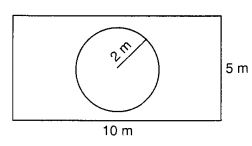Solution: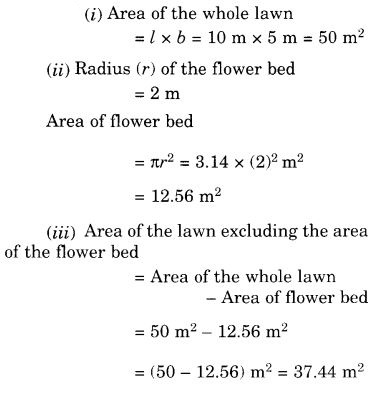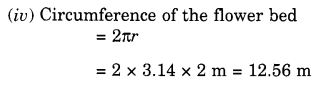Question 10.
In the following figures, find the area of the shaded portions.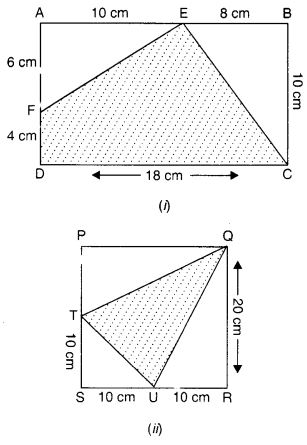Solution: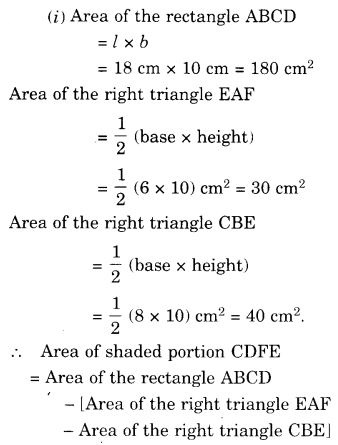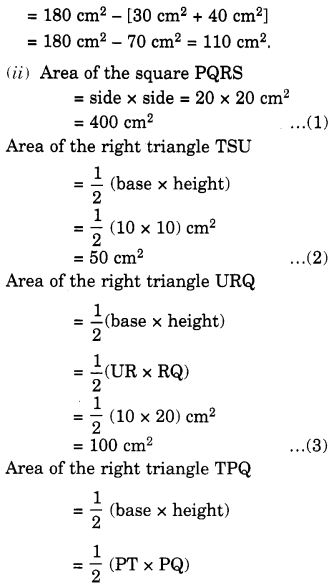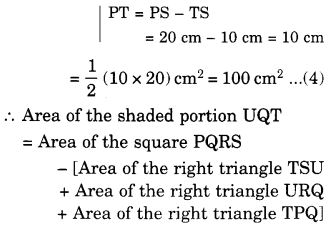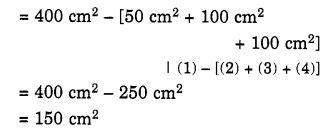Question 11.
Find the area of the quadrilateral ABCD.
Here, AC = 22 cm, BM = 3 cm, DN = 3 cm, and BM ⊥ AC, DN ⊥ AC.
Solution:
Area of the quadrilateral ABCD = Area of the triangle ABC + Area of the triangle ADC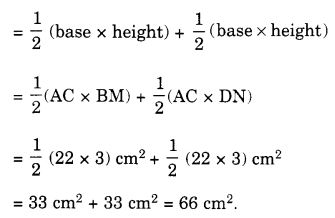We hope the NCERT Solutions for Class 7 Maths Chapter 11 Perimeter and Area Ex 11.4 help you. If you have any query regarding NCERT Solutions for Class 7 Maths Chapter 11 Perimeter and Area Ex 11.4, drop a comment below and we will get back to you at the earliest.